(Artificial) Neural Networks (ANN)

By Prof. Seungchul Lee
http://iai.postech.ac.kr/
Industrial AI Lab at POSTECH

# 1. Recall Supervised Learning Setup¶

Perceptron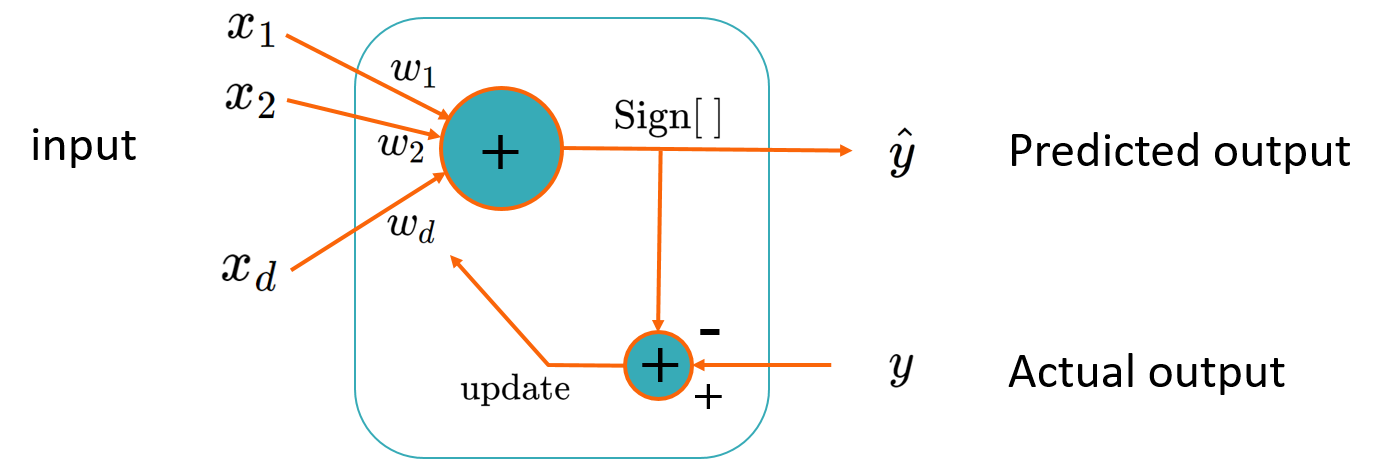XOR Problem

• Minsky-Papert Controversy on XOR
• not linearly separable
• limitation of perceptron
$x_1$ $x_2$ $x_1$ XOR $x_2$
0 0 0
0 1 1
1 0 1
1 1 0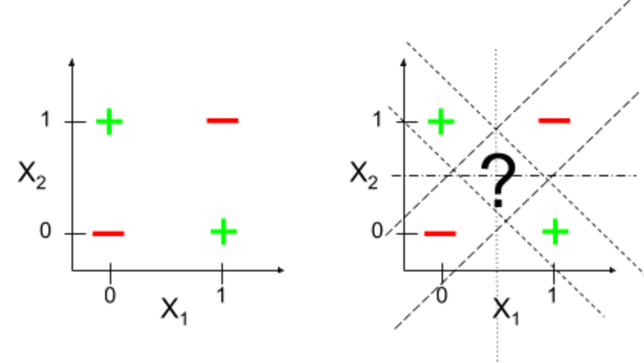# 2. From Perceptron to Multi-Layer Perceptron (MLP)¶

## 2.1. Perceptron for $h_{\omega}(x)$¶

• Neurons compute the weighted sum of their inputs

• A neuron is activated or fired when the sum $a$ is positive

\begin{align*} a &= \omega_0 + \omega_1 x_1 + \omega_2 x_2 \\ \\ \hat{y} &= g(a) = \begin{cases} 1 & a > 0\\ 0 & \text{otherwise} \end{cases} \end{align*}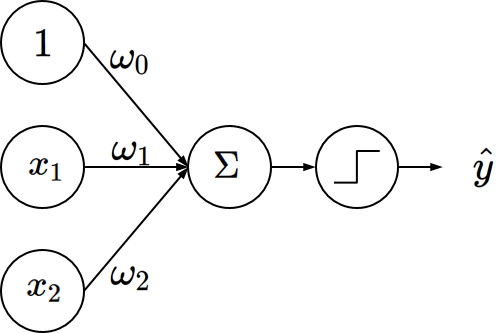• A step function is not differentiable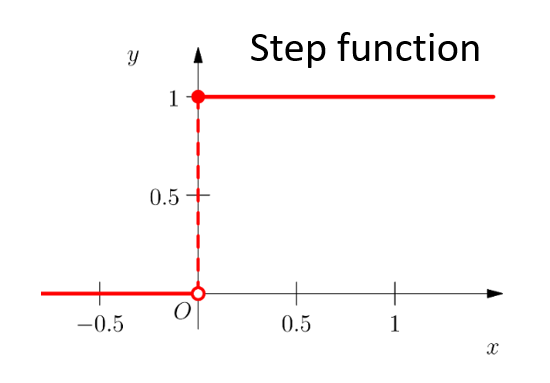• One layer is often not enough
• One hyperplane

## 2.2. Multi-layer Perceptron = Artificial Neural Networks (ANN)¶

Multi-neurons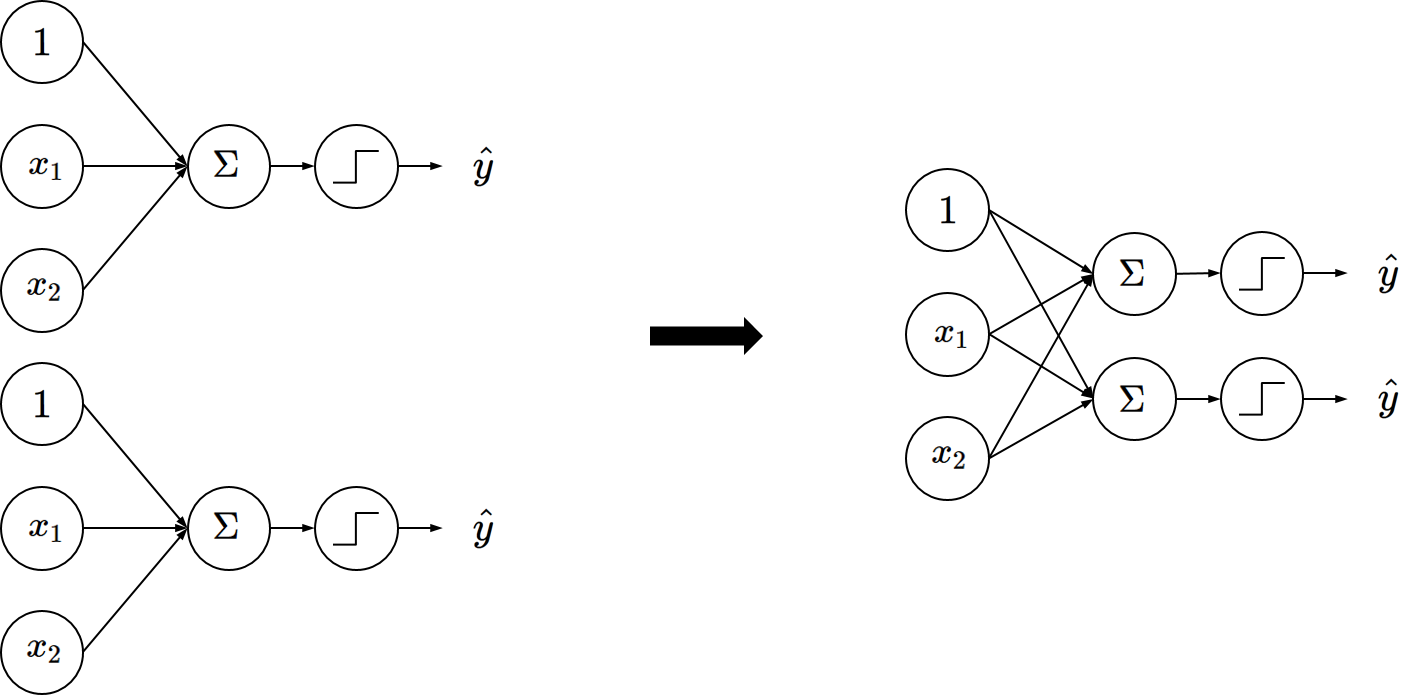Differentiable activation function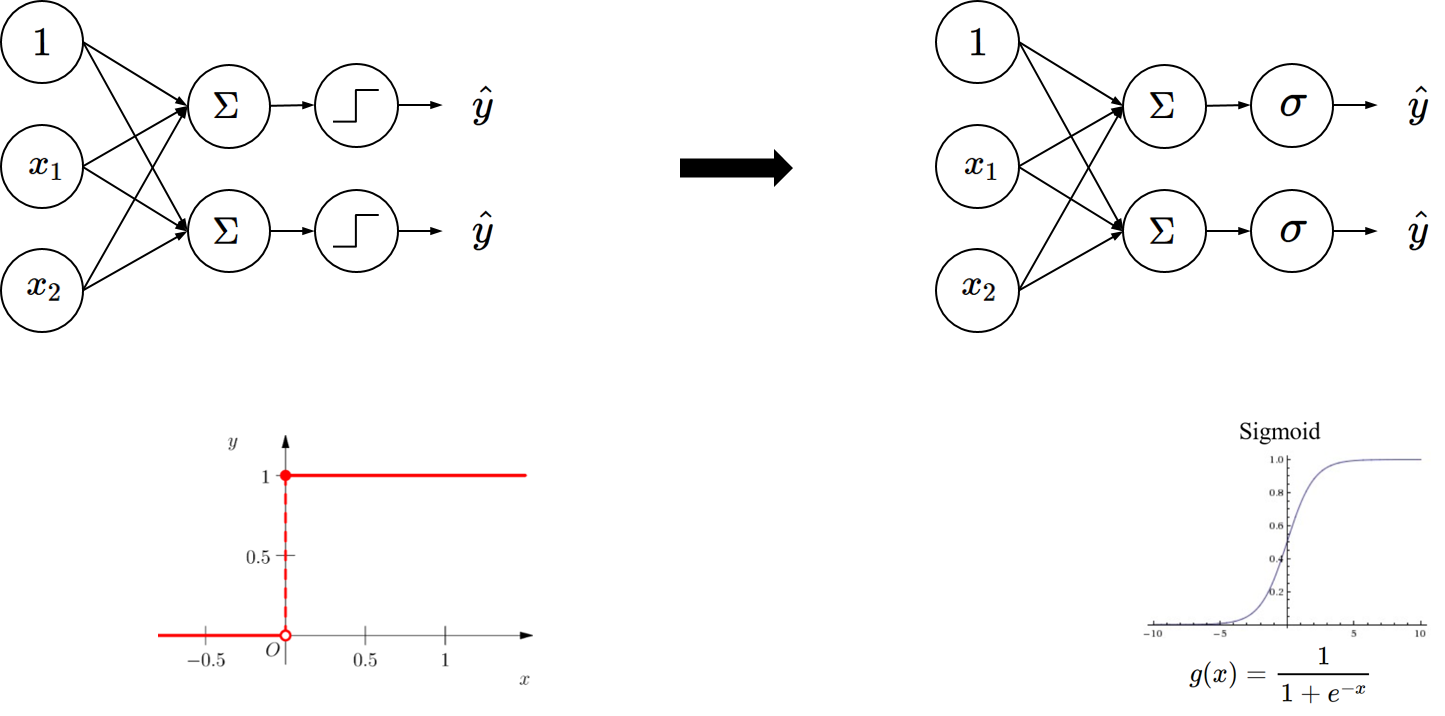In a compact representation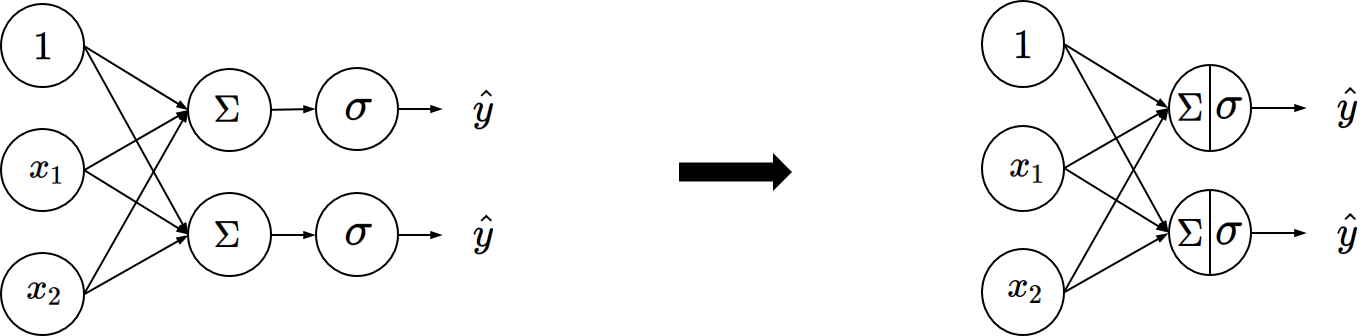Multi-layer perceptron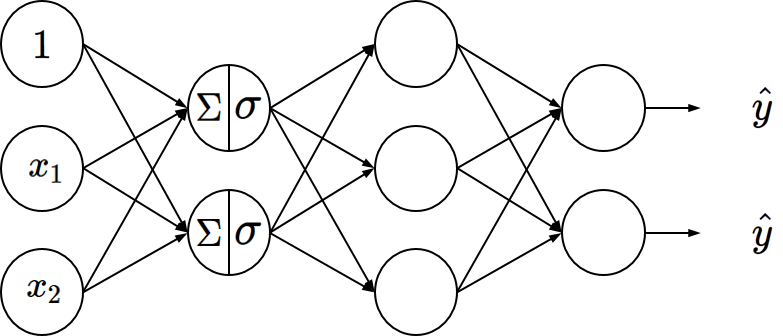## 2.3. Another Perspective: ANN as Kernel Learning¶

In :
%%html
width="420" height="315" frameborder="0" allowfullscreen></iframe></center>


We can represent this “neuron” as follows:

• The main weakness of linear predictors is their lack of capacity. For classiﬁcation, the populations have to be linearly separable.

• The XOR example can be solved by pre-processing the data to make the two populations linearly separable.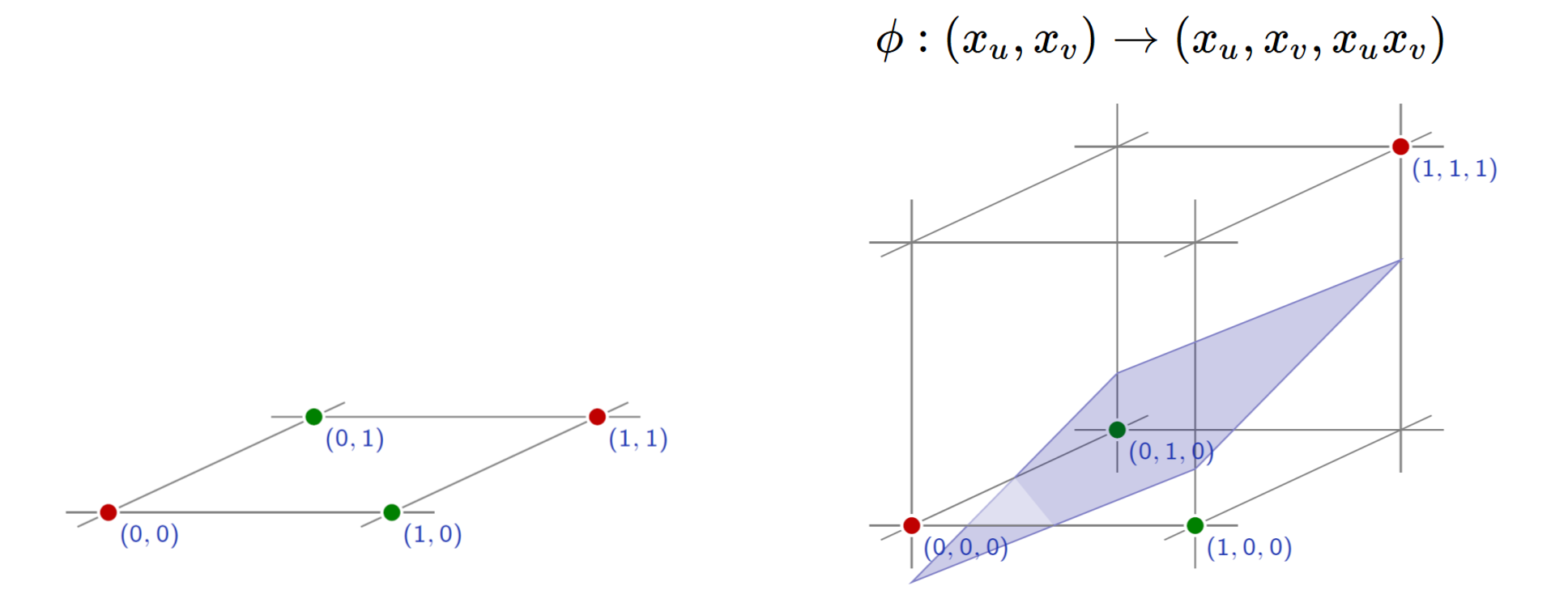Kernel

Often we want to capture nonlinear patterns in the data

• nonlinear regression: input and output relationship may not be linear
• nonlinear classification: classes may note be separable by a linear boundary

Linear models (e.g. linear regression, linear SVM) are not just rich enough

• by mapping data to higher dimensions where it exhibits linear patterns
• apply the linear model in the new input feature space
• mapping = changing the feature representation

Kernels: make linear model work in nonlinear settings

Kerenl + Neuron

• Nonlinear mapping can be represented by another neurons